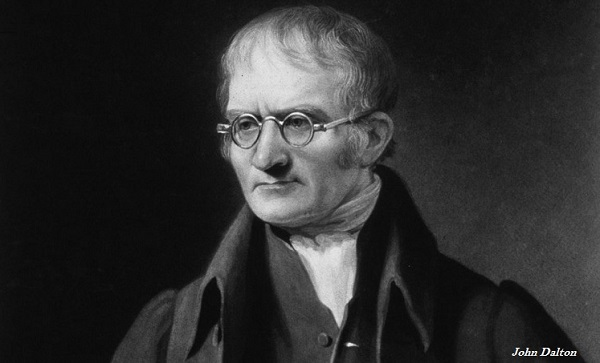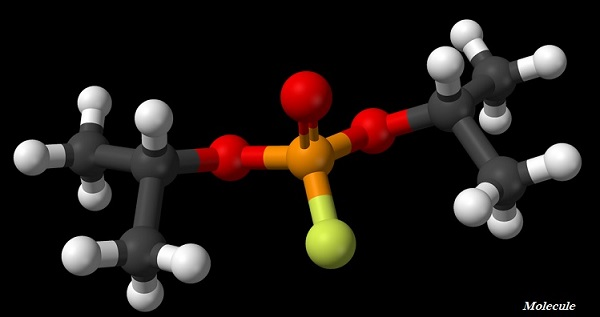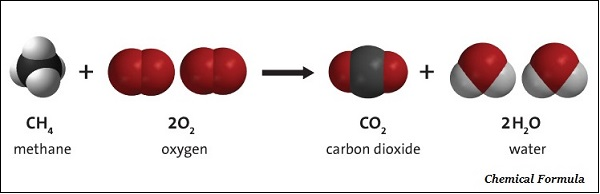# Chemistry - Atoms & Molecules

## Introduction

• Around 500 BC, an Indian Philosopher Maharishi Kanad, first time postulated the concept of indivisible part of matter and named it ‘pramanu.’

• In 1808, John Dalton used the term ‘atom’ and postulated the atomic theory to the study of matter.## Dalton’s Atomic Theory

• According to Dalton’s atomic theory, all matter, whether an element, a compound or a mixture is composed of small particles called atoms.

• According to Dalton’s atomic theory, all matters, whether they are elements, compounds, or mixtures, are composed of small particles known as atoms.

### Salient features of Dalton’s Atomic Theory

• All matter is made of very miniscule particles known as atoms.

• Atom is an indivisible particle, which cannot be created or destroyed through chemical reaction.

• All atoms of an element are identical in mass and chemical properties whereas, atoms of different elements have different masses and chemical properties.

• To form a compound, atoms are combined in the ratio of small whole numbers.

• In a given compound, the relative number and kinds of atoms are constant.

## Atomic Mass

• The mass of an atom of a chemical element; it is expressed in atomic mass units (symbol is u).

• The atomic mass is roughly equivalent to the number of protons and neutrons present in the atom.

• One atomic mass unit is a mass unit equal to the exactly one-twelfth (1/12th) the mass of one atom of carbon-12 and the relative atomic masses of all elements have been calculated with respect to an atom of carbon-12.

## Molecule

• The smallest particle of an element or a compound, which is capable to exist independently and shows all the properties of the respective substance.• A molecule, normally, is a group of two or more atoms which are chemically bonded together.

• Atoms of the same element or of different elements can join (with chemical bond) together to form molecules.

• The number of atoms that constitute a molecule is known as its atomicity.

## Ion

• A charged particle is known as ion; it could be either negative charge or positive charge.

• The positively charged ion is known as a ‘cation’.

• The negatively charged ion is known as an ‘anion.’

## Chemical Formulae

• A chemical formula of a compound demonstrations its constituent elements and the number of atoms of each combining element.• The chemical formula of a compound is the symbolic representation of its Composition.

• The combining capacity of an element is known as its ‘valency.’

## Molecular Mass

• The molecular mass of a substance is calculated by taking the sum of the atomic masses of all the atoms in a molecule of respective substance. For example, the molecular mass of water is calculated as −

• Atomic mass of hydrogen = 1u

• Atomic mass of oxygen = 16 u

• The water contains two atoms of hydrogen and one atom of oxygen.

• Molecular Mass of Water is = 2 × 1+ 1×16 = 18 u (u is the symbol of molecular mass).

## Formula Unit Mass

• The formula unit mass of a substance is calculated by taking the sum of the atomic masses of all atoms in a formula unit of a compound.

• The number of particles (atoms, molecules, or ions) present in 1 mole of any substance is fixed, and its value always calculated as 6.022 × 1023.

• In 1896, Wilhelm Ostwald had introduced the concept of ‘mole;’ however, mole unit was accepted to provide a simple way of reporting a large number in 1967.

## Law of Conservation of Mass

• During a chemical reaction, sum of the masses of the reactants and products remains unchanged, which is known as the ‘Law of Conservation of Mass.’

## Law of Definite Proportions

• In a pure chemical compound, its elements are always present in a definite proportion by mass, which is known as the ‘Law of Definite Proportions.’